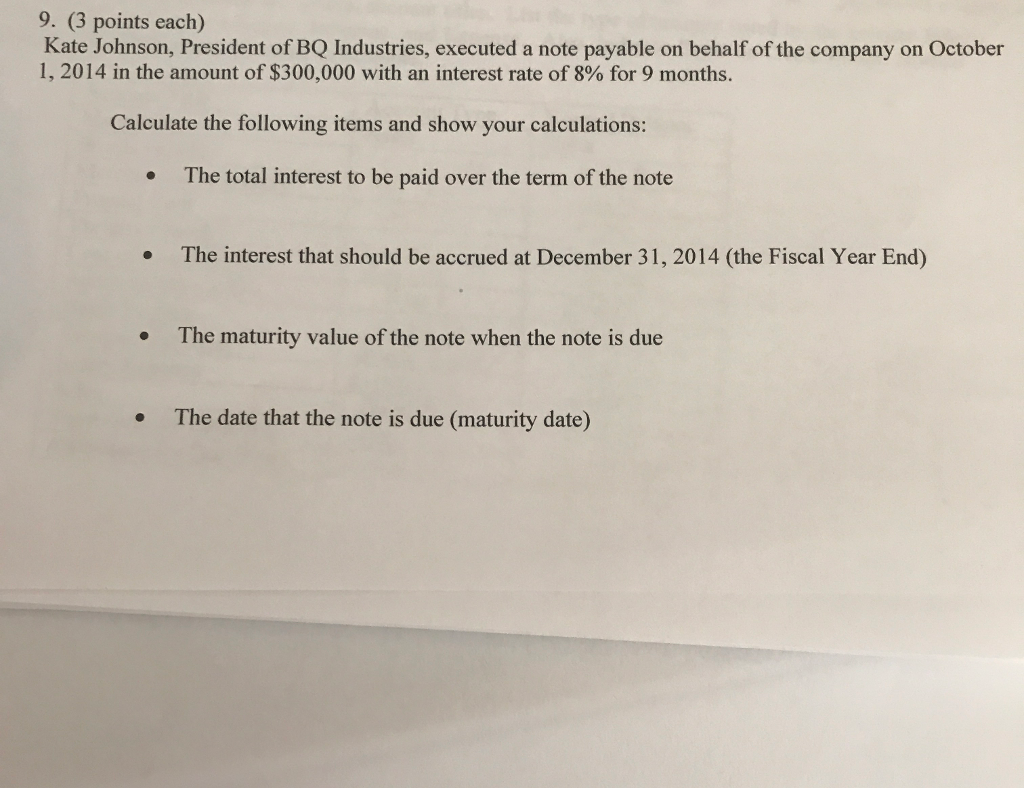# Question & Answer: Kate Johnson, President of BQ Industries, executed a note payable on behalf of the company on October 1,…..Kate Johnson, President of BQ Industries, executed a note payable on behalf of the company on October 1, 2014 in the amount of \$300,000 with an interest rate of 8% for 9 months. Calculate the following items and show your calculations: The total interest to be paid over the term of the note The interest that should be accrued at December 31, 2014 (the Fiscal Year End) The maturity value of the note when the note is due The date that the note is due (maturity date)

Don't use plagiarized sources. Get Your Custom Essay on
Question & Answer: Kate Johnson, President of BQ Industries, executed a note payable on behalf of the company on October 1,…..
GET AN ESSAY WRITTEN FOR YOU FROM AS LOW AS \$13/PAGE

1) Total interest = Principal amount × Rate × Time

Total interest = \$300,000 × 8% × 9/12

Total interest = \$18,000

2) Accrued Interest = Principal amount × Rate × Time

Accrued Interest = \$300,000 × 8% × 3/12

Accrued Interest = \$6,000

3) Maturity Value = Principal + Interest

Maturity Value = \$300,000 + \$18,000

Maturity Value = \$318,000

4) Issuance date = Oct 1, 2014

Number of months = 9

Due Date = July 1, 2015